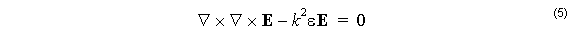The time independent Maxwell curl equations with a positive time convention (ej ω t )
are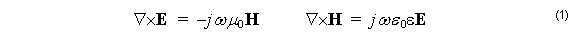and the divergence equations are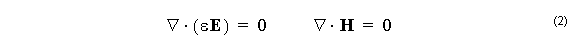The factor j does not suit the current purpose, so make the substitution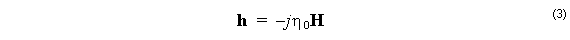where n0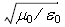is the free space impedance, so that h and E have the same

units. Then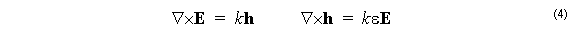where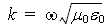. Eliminating the h gives the electric wave equation